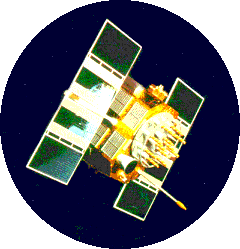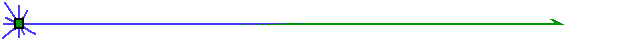# Satellites Everywhere...

### "Watching over You"Homework Quiz

Lesson Objectives:

• Be able to show how Kepler's third law (FOR A CIRCULAR ORBIT) can be derived using Newton's second law and the law of gravitation.
• Be able to calculate the period and altitude of geostationary satellites.
• Know and understand how Kepler's three laws of planetary motion are applications of Newton's second law and conservation of angular momentum.
• Be able to apply Newton's second law and conservation of angular momentum to ANALYZE problems involving the orbit of an object about a star or planet.
• Be able to compare different kinds of satellite orbits, and understand why certain types of satellites require certain orbits.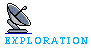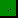NASA Orbital TheoryPolar Orbits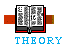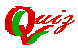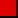OrbitsKepler's Laws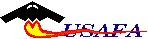Click here to return to the Mission Planning Page